# [Homology] Count the number of holes in data with Python

Jupyter Notebook is a convenient tool that allows you to check the calculation results on the way while writing a program. Another advantage of using Google Colaboratory is that you can calculate regardless of your environment.

Topological data analysis is one of the methods of data analysis that has become a hot topic these days. This is an analysis method that captures data as a form and counts how many features such as holes are in it. First, as the name implies, we will discuss Topology.

# What is Topology?

According to wikipedia, "Topology is a property (topological property or phase" that can be maintained even if some form (shape, or "space") is continuously deformed (stretched or bent, but not cut or pasted). It focuses on invariants). It is said.

Many of you may have heard that "coffee cups" and "doughnuts", which are often used to explain Topology, are the same from a Topological point of view.Quoted from wikipedia Topology

From this figure, you can see that the "coffee cup" and "doughnut" can be deformed by bending and stretching without cutting and pasting. This means that the number of holes, which is a topological invariant, has one thing in common. In this article, we calculated Homology, which counts the number of holes in a 2D figure.

# Homology calculation procedure

The specific calculation of homology is Input: Data coordinates, data radius Output: Number of holes in the data I will do it as.

# Enter data

Enter the data you want to calculate. This time, the calculation was performed using randomly created data.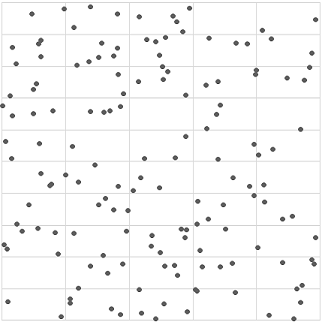import os
import random as rd
import numpy as np
import copy
#Point cloud data creation
#Data dimension
dim = 2
#The number of data
data_size = 500
sim_r = 0.08
def make_point_cloud(dim,size):
arr = np.random.rand(size, dim).astype(np.float64)
return arr
data = make_point_cloud(dim,data_size)


Then, in order to calculate the number of holes, we calculate the concatenation in which the data are connected.

# Calculation of concatenation

Since the data is just for sitting, the figure below gives the data a radius.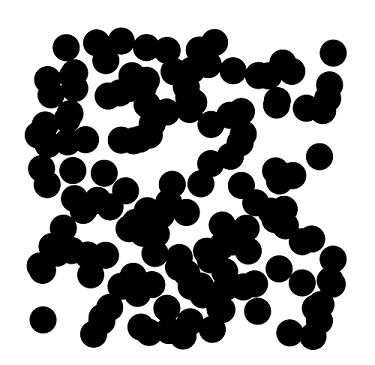def distance(data1,data2):
r = data1 - data2;
r = np.sqrt(np.sum(np.power(r,2.0)))
return r
def make_path_matrix(data,r):
size ,data_dim = data.shape
path_matrix = [[0 for j in range(size)]for i in range(size)]
for i in range(size):
for j in range(size):
if distance(data[i],data[j]) <= 2*r:
path_matrix[i][j] = 1;
return path_matrix
pathM = make_path_matrix(data,sim_r)
#Deta arrival flag not reached: 0 reached: 1
flaglist = [0 for i in range(data_size)]
#A function that recursively searches an adjacency matrix
def visit(i,path):
flaglist[i] = 1
for j in range(data_size):
if(path[i][j] == 1 and flaglist[j] == 0):
visit(j,path)
#Connected body
K = []
for i in range(data_size):
#Save one of the conjugates
comp = [];
#Save flaglist before search
flaglistsave = copy.deepcopy(flaglist);
#Check if it has been searched
if flaglist[i] == 0:
#Search when not searched
visit(i,pathM);
#Check the search flag list
for flagindex in range(data_size):
#Confirm the searched and newly searched points
if flaglist[flagindex] == 1 and flaglistsave[flagindex] == 0:
comp.append(flagindex);
#Added to the set of concatenations
K.append(comp);



It can be seen that by giving the data a radius, it can be divided into connected bodies in which circles are connected. Next, we examine the smallest simple substance contained in the conjugate.

# Creating a single unit

A simple substance is the smallest unit that makes up a connected body, and a simple substance is a point, line, or surface.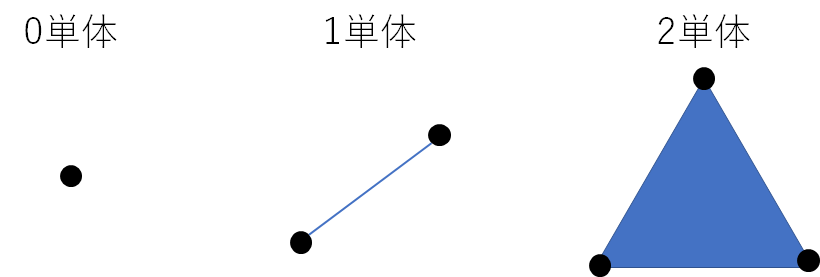When there are only points, it is called 0 unit, a line is called 1 unit, and a triangular surface is called 2 unit. This time, it is limited to 2 units to count 2D holes, but in 3D, the tetrahedron becomes 3 units and is defined in higher dimensions.

In the configuration of 2 units, as shown in the figure below, there are cases where each piece of the triangle constitutes 1 unit but does not constitute 2 units.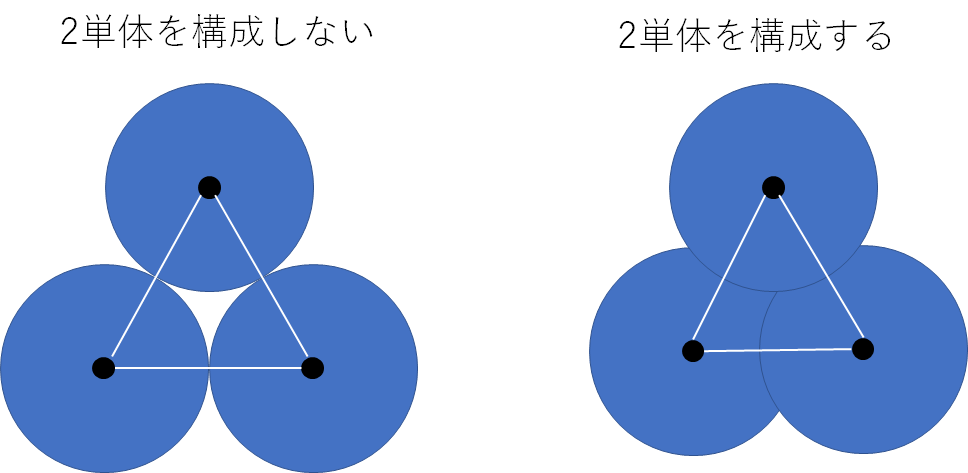~~ It seems that you can use the alpha complex, which has a high calculation speed, but I don't know, so I calculated it with the check complex ~~

#2 Check the configuration of a single unit and improve
def checksim2(point,r):
a = point;
b = point;
c = point;
da = distance(b,c)
db = distance(a,c)
dc = distance(b,a)
#Check if each of the three sides constitutes one unit
sim1flag = da <(2*r) and db<(2*r) and dc<(2*r)
#Heron's formula
ss = (da + db + dc)/2
#print(ss * (ss - da)*(ss - db)*(ss - dc))
S = np.sqrt(ss * (ss - da)*(ss - db)*(ss - dc))
MaxS = np.power(r,2) * np.sin(np.pi/6)*np.cos(np.pi/6)/2*3
#Area confirmation
Sflag = S<MaxS
#Circumscribed circle judgment
cosC = (np.power(da,2) + np.power(db,2) - np.power(dc,2))/(2*da*db)
sinC =np.sqrt(1 - np.power(cosC,2))
outerflag = 2*sinC*sim_r > dc
if (Sflag and sim1flag) or (outerflag and sim1flag):
return 1
else:
return 0
#Number of articulations
betti0 = len(K)
#1 Single list
sim1list = [[] for i in range(betti0)]
#2 Single list
sim2list = [[] for i in range(betti0)]

#Extract a conjugate with only one 1 and 2 simple substances
for i in range(betti0):
if len(K[i]) <4 and len(K[i]) != 1:
if len(K[i]) == 2:
sim1list[i] = copy.deepcopy([K[i]])
if len(K[i]) == 3 and checksim2([data[K[i]],data[K[i]],data[K[i]]],sim_r) == 1:
sim2list[i] = copy.deepcopy([K[i]])
#Extract 1 simple substance from the conjugate
for b in range(betti0):
if len(K[b]) > 2:
for i in range(1,len(K[b]),1):
for j in range(0,i,1):
if pathM[K[b][i]][K[b][j]] == 1:
sim1list[b].append([K[b][i],K[b][j]])
#Extract 2 simple substances from the conjugate
for b in range(betti0):
if len(K[b]) > 3:
for i in range(2,len(K[b]),1):
for j in range(1,i,1):
for k in range(0,j,1):
l = [data[K[b][i]],data[K[b][j]],data[K[b][k]]]
if checksim2(l,sim_r) == 1:
sim2list[b].append([K[b][i],K[b][j],K[b][k]])



By counting the simple substances in the concatenation, a set containing a finite number of simple substances is created. Next, a map that transforms a simple substance into a simple substance of another dimension, a boundary map, will be described.

# Boundary map

A boundary map is a map that transforms a simple substance into a small one-dimensional simple substance.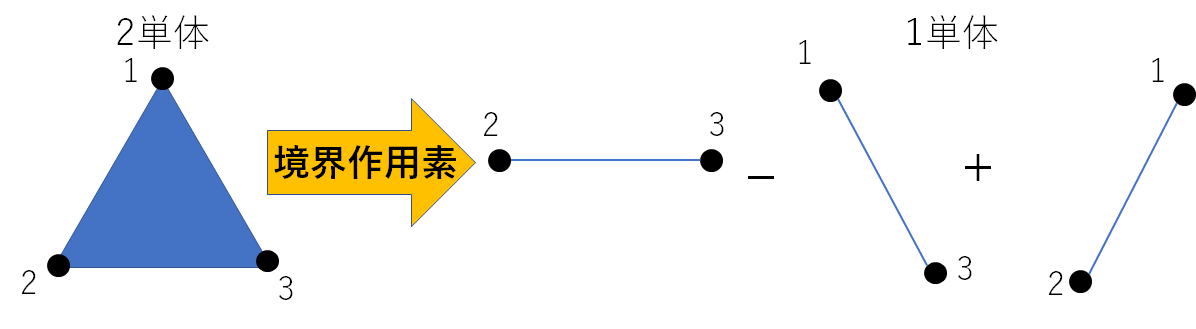As shown in the figure, it is a mapping that converts from 2 simple substances to the alternating sum of 1 simple substance. The concrete map is as follows

\langle 1,2,3 \rangle = \langle 2,3 \rangle - \langle 1,3 \rangle + \langle 1,2 \rangle



The number removed from the original simple substance is a mapping that takes the sum by changing the sign of the number from the front with even and odd numbers. The boundary map that transfers from 2 units to 1 unit contained in the conjugate is called the secondary boundary map, and the boundary map that transfers from 1 unit to 0 units is called the primary boundary map. The obtained second-order boundary map and first-order boundary map are represented as representation matrices. Next, the calculation for counting the number of holes from this boundary operator will be described.

#Sort concatenation, 1 unit, 2 unit
cmp = [sorted(l, reverse=True) for l in K]
K = copy.deepcopy(cmp)
del cmp
cmp = [[ sorted(s, reverse=True) for s in l]for l in sim1list]
sim1list = copy.deepcopy(cmp)
del cmp
cmp = [[ sorted(s, reverse=True) for s in l]for l in sim2list]
sim2list = copy.deepcopy(cmp)
del cmp
#Primary boundary operator
boundary1 = [[] for _ in range(betti0)]
for b in range(betti0):
if len(sim1list[b]) > 0:
M = np.ones((len(K[b]),len(sim1list[b])),dtype=np.int8)
for i in range(len(K[b])):
for j in range(len(sim1list[b])):
if len(K[b]) > 1:
if sim1list[b][j] == K[b][i]:
M[i][j] = 1
else:
if sim1list[b][j] == K[b][i]:
M[i][j] = -1
else:
M[i][j] = 0
else:
if sim1list[b][j] == K[b]:
M[i][j] = 1
else:
if sim1list[b][j] == K[b]:
M[i][j] = -1
else:
M[i][j] = 0
boundary1[b] = copy.deepcopy(M)
boundary2 = [[] for _ in range(betti0)]
for b in range(betti0):
if len(sim2list[b]) > 0:
M = np.ones((len(sim1list[b]),len(sim2list[b])),dtype=np.int8)
for i in range(len(sim1list[b])):
for j in range(len(sim2list[b])):
if [sim2list[b][j],sim2list[b][j]] == sim1list[b][i]:
M[i][j] = 1
else:
if [sim2list[b][j],sim2list[b][j]] == sim1list[b][i]:
M[i][j] = -1
else:
if [sim2list[b][j],sim2list[b][j]] == sim1list[b][i]:
M[i][j] = 1
else:
M[i][j] = 0
boundary2[b] = copy.deepcopy(M)


# Count the number of holes

The number of holes in the data is in the area shown in the following figure.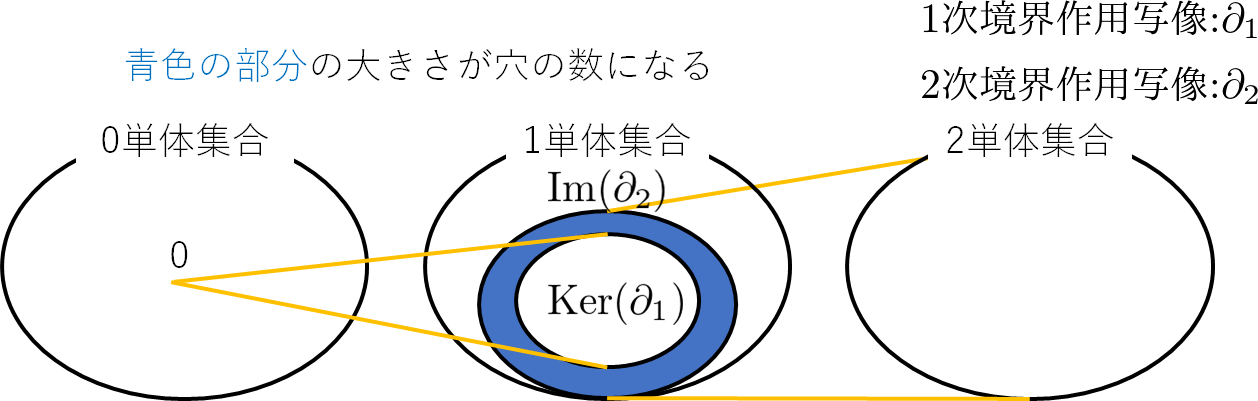The image of the secondary boundary map $\ partial_2$ and the core of the primary boundary map $\ partial_1$ are each a convertible group, and it seems that the number of holes can be obtained from the dimension of the quotient group. I don't know the exact details, so the formula calculated this time is shown below.

B_1 = dim(Ker(\partial_1)) - dim(Im(\partial_2))\\
B_1 = (dim(C_1) - rank(\partial_1)) - rank(\partial_2)


$dim (C_1)$ is the number of 1 element contained in the concatenation

#Variable that counts the number of primary Betti
betti1 = 0
for b in range(betti0):
rank1 = 0
rank2 = 0
#Confirmation of the presence or absence of primary boundary operators
if len(boundary1[b]) != 0:
rank1 = GPU_rank(boundary1[b])
if len(boundary2[b]) != 0:
rank2 = GPU_rank(boundary2[b])
betti1 += len(sim1list[b]) - rank1 - rank2
print("Concatenated number",b,"  ",len(sim1list[b]),"-",rank1,"-",rank2,"=",len(sim1list[b]) - rank1 - rank2)
print("The primary Betti number is",betti1,"Becomes")


# Calculation result

When you visualize the data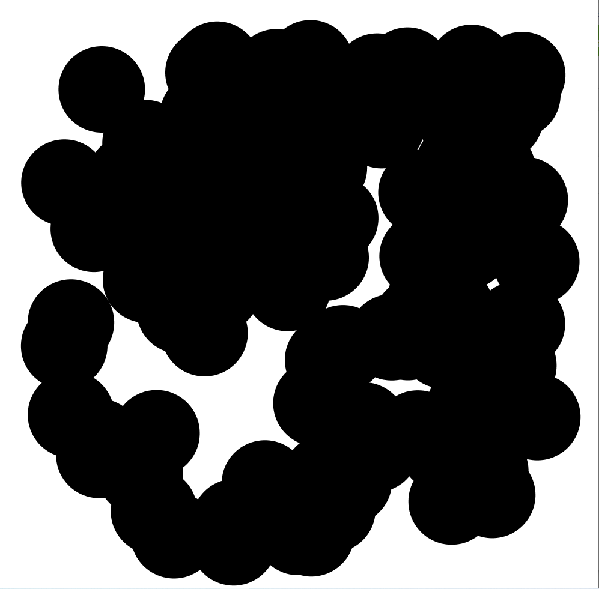It can be seen that there are five white holes in the figure. In addition, the result of performing the Homology calculation program with this data

Concatenated number 0 414- 99 - 310 = 5
The primary Betti number is 5.


You can see that the number of holes was counted.

# Data visualization

Processing was used for data visualization. The program is here.

String lin;
String linR;
int ln;
static final int img_size = 800;
float r;
String lines[];
String linesR[];
void setup() {
ln = 0;
//r = 0.1;
linR = linesR;//Stores the ln line
String [] coR = split(linR,',');
if (coR.length == 1){
r = float(coR);
}
background(#FFFFFF);
size(1000,1000);
println(r);
for(ln = 0;ln < lines.length;ln++){
lin = lines[ln];//Stores the ln line
String [] co = split(lin,',');

if (co.length == 2){
float x = float(co);
float y = float(co);
fill(#000000);
noStroke();
ellipse(x*img_size + 100,
1000 -(y*img_size + 100),
2*r*img_size,
2*r*img_size);
}
}
}

void draw() {

}


Save the coordinate data as pointcloud.csv and the radius value as r.csv.